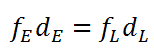# Lever Force Calculator

This CalcTown calculator calculates the lever force required to balance a lever, given the distance of effort and load from the fulcrum.

N
m
m

#### Result

Nwhere

fE: Effort force
dE: Distance of effort force to fulcrum
fL: Load force
dL: Distance of load force to fulcrum